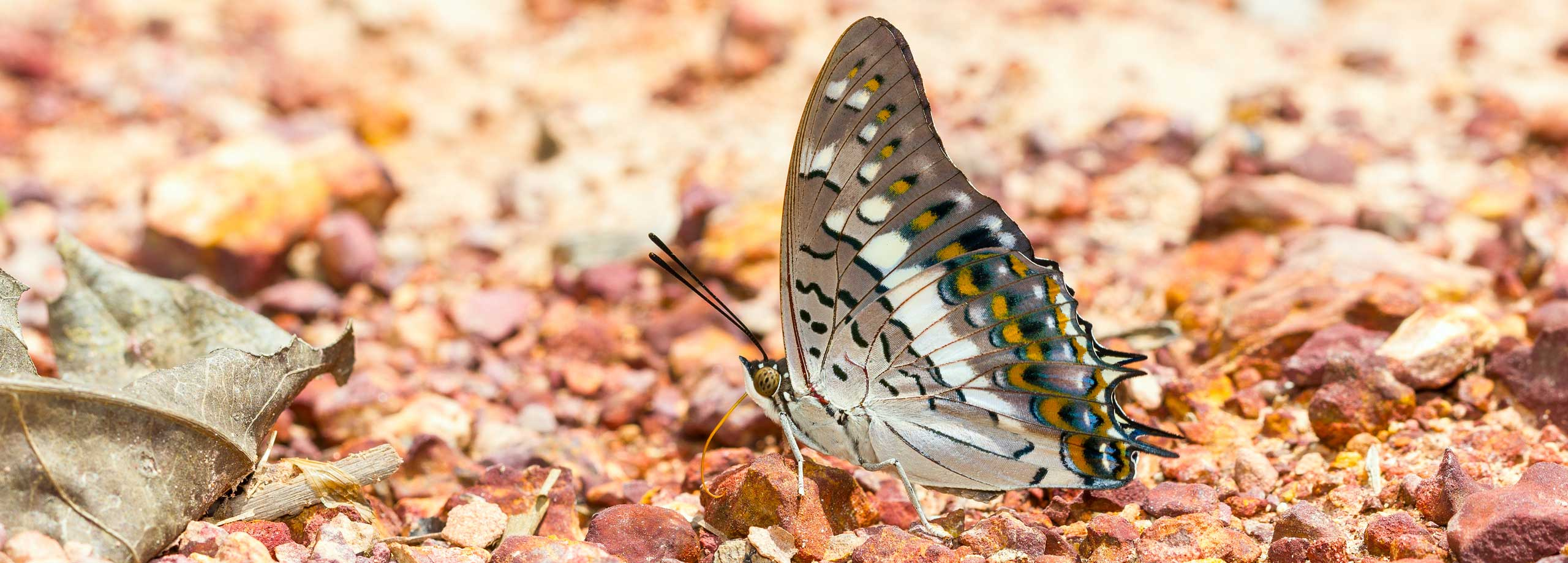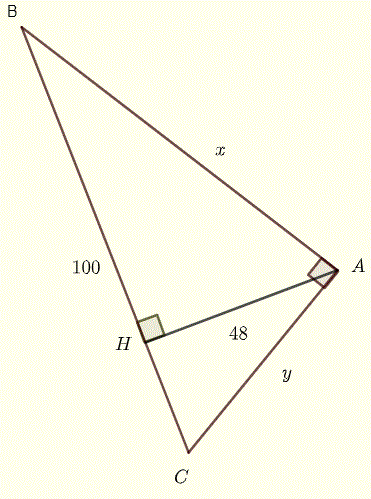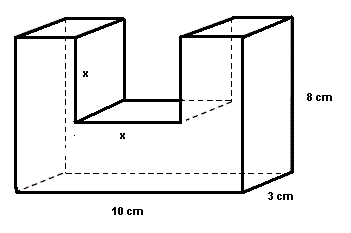# EmSAT Math Practice Questions - Sample 1

EmSAT math practice questions are presented along with their answers at the bottom of the page. There are four groups of questions: algebra, geometry, statistics and probabilities, and calculus.
The detailed solutions to the questions below will be posted later.

## Algebra Questions to Practice for the EmSAT

     
1. Question 1

What is the solution set to the equation   $4 = -(x +2)(x-1)$?

1. $\left\{-\frac{1}{2}-i\dfrac{\sqrt{7}}{2} \quad , \quad \frac{1}{2}+i\dfrac{\sqrt{7}}{2} \right\}$
2. $\{-2 , 1\}$
3. $\left\{\frac{1}{2}-i\dfrac{\sqrt{7}}{2} \quad , \quad \frac{1}{2}+i\dfrac{\sqrt{7}}{2} \right\}$
4. $\left\{-\frac{1}{2}-i\dfrac{\sqrt{7}}{2} \quad , \quad -\frac{1}{2}+i\dfrac{\sqrt{7}}{2} \right\}$

2. Question 2

Find all solutions to the equations   $9^{-x\left(-x+5\right)}\:= \dfrac{1}{3^{-12}}$.

1. $\{6,2\}$
2. $\{-1 , -2\}$
3. $\{-1 , 6\}$
4. $\{6,1\}$

3. Question 3

Write the expression   $\dfrac{1}{3 + \sqrt{-4}}$ as a complex number of the form $a + i b$.

1. $\dfrac{3}{13}- i \dfrac{2}{13}$

2. $\dfrac{1}{3}- i \dfrac{1}{2}$

3. $\dfrac{3}{13} + i \dfrac{2}{13}$

4. $\dfrac{1}{3} + i \dfrac{1}{2}$

4. Question 4

The graph the complex number   $-2(4 - i)$ is in quadrant

1. $I$
2. $III$
3. $IV$
4. $II$

5. Question 5

Ahmed rented a car for two days and he paid a total of 261 AED. He was charged a fixed fee of 80 AED per day plus 20 fils for every kilometer traveled.
What is the total distance traveled? (Give your answer to the nearest kilometer)

1. $250$ kilometers
2. $505$ kilometers
3. $1305$ kilometers
4. $905$ kilometers

6. Question 6

Solve for $x$ and $y$ the following system of equations.
$\begin{cases} \dfrac{x}{2}+\dfrac{y}{2}=1\\\\ \dfrac{x}{3}+\dfrac{y}{4}=2 \end{cases}$

1. $(3 , -2)$
2. $(-18,-16)$
3. $(18,-16)$
4. $(-16,18)$

7. Question 7

Simplify.
$\dfrac{x^2 - 4y^2}{2y - x}$

8. Question 8

Simplify the expression   $\sqrt{200} + \sqrt{32}$.

1. $2 \sqrt{58}$
2. $14 \sqrt{2}$
3. $6 \sqrt{2}$
4. $2 \sqrt{14}$

9. Question 9

We suppose that the point with coordinates $(a , - 3)$ is on the graph of the curve $y = x(x-2)$. Find the constant $a$ if possible.

1. $a = 3$
2. $a = 1$
3. No real value of $a$, which satisfies the above conditions, exist.
4. $a = -3$

10. Question 10

The solution set of the equation   $log_4(x) - log_4(x+10) = -log_4(x-2)$ is

1. $\{-2,5\}$
2. $\{-2\}$
3. $\{2,5\}$
4. $\{5\}$

11. Question 11

Find all the solutions of the equation   $2 - \dfrac{1}{x(x+1)} = \dfrac{3}{x+1}$.

1. $\{-1/2\}$
2. $\{1\}$
3. $\{-1/2,1\}$
4. $\{1/2 , - 1\}$

12. Question 12

Find   $f^{-1}(-2)$ if   $f(x) = \sqrt{x + 2} - 4$.

1. $2$
2. $-2$
3. $-4$
4. $0$

13. Question 13

Which of the following is equivalent to the expression $sin(4x)$ for all values of $x$?

1. $4 \sin(x)$
2. $4 \sin(x) \cos (x) \cos(2x)$
3. $2 \sin(2x)$
4. $sin(x) + 4$

14. Question 14

What is the magnitude of vector $\overrightarrow v$ given by $\overrightarrow v = 2 \overrightarrow{v_1} - 3 \overrightarrow {v_2}$ where   $\overrightarrow{v_1} = <1,-2>$ and   $\overrightarrow{v_2} = <-2,4>$?

1. $2 \sqrt {6}$
2. $8$
3. $8 \sqrt {5}$
4. $6$

15. Question 15

A factory produced 2000 toys two years ago and 2400 toys this year. If we assume that the production varies linearly with the time, what will be the production of the factory in four years?

1. $3200$   toys
2. $2800$   toys
3. $2600$   toys
4. $3000$   toys

16. Question 16

Find the solution set of the inequality $\quad x + 4 \le \dfrac{3}{x+2}$.

1. $(-\infty ,-5]$
2. $(-\infty ,-5] \cup (-2,-1)$
3. $(-\infty ,-5] \cup (-2,-1]$
4. $(-\infty ,-5) \cup (-2,-1]$

17. Question 17

Simplify: $(-x+2)(x-1) - ( x^2 - 2x +1)$

1. $-2x^2+x-1$
2. $-2x^2 - x-1$
3. $2x^2+5x-3$
4. $-2x^2+5x-3$

18. Question 18

Factor   $3x^2 + 4x - 4$ completely.

19. Question 19

Find two positive real numbers such that their difference is $2$ and their product is $99$.

20. Question 20

Solve the equation $\quad x y = \dfrac{y - 1}{x - 1}$ for $y$.

21. Question 21

The sum of three real numbers $x , y$ and $z$ is equal to 96. The sum of $y$ and $z$ is equal to $74$ and the difference $z - y$ is equal to $12$. Find the three numbers.

22. Question 22

Which of the following equation could be the equation to the third degree polynomial $P$ whose graph is shown below?1. $P(x) = (x-2) (x+1) (x+3)$
2. $P(x) = -0.5 (x+2) (x-1) (x-3)$
3. $P(x) = 0.5 (x-2) (x+1) (x+3)$
4. $P(x) = -0.5 (x-2) (x+1) (x-3)$

23. Question 23

Let $f(x) = x^2 - 1$ and $g(x) = \dfrac{1}{x-2}$; find $(f_o g)(0)$.

1. $-\dfrac{3}{4}$
2. $-1$
3. $-\dfrac{1}{2}$
4. $0$

24. Question 24

Mansour drunk a cup of coffee with 120 milligrams of caffeine at 8:00 am. After five hours, the caffeine in decreased exponentially to half the initial amount. How much caffeine is left in Mansour's body at 6:00 pm?

1. $60$ milligrams
2. $15$ milligrams
3. $45$ milligrams
4. $30$ milligrams

25. Question 25

Write the system of equations $\begin{cases} -2x + y - z = 1\\\\ 5x - y = -3\\\\ -2x + 2z - 4 y = 0 \end{cases}$ in matrix form.

1. $\begin{bmatrix} -2 & 1 & -1 \\ 5 & -1 & 1 \\ -2 & - 4 & 2 \end{bmatrix} \cdot \begin{bmatrix} x\\ y\\ z \end{bmatrix} = \begin{bmatrix} 1 \\ -3 \\ 0 \end{bmatrix}$

2. $\begin{bmatrix} -2 & 1 & -1 \\ 5 & -1 & 0 \\ -2 & - 4 & 2 \end{bmatrix} \cdot \begin{bmatrix} x\\ y\\ z \end{bmatrix} = \begin{bmatrix} 1 \\ -3 \\ 0 \end{bmatrix}$

3. $\begin{bmatrix} -2 & 1 & -1 \\ 5 & -1 & 0 \\ -2 & 2 & -4 \end{bmatrix} \cdot \begin{bmatrix} x\\ y\\ z \end{bmatrix} = \begin{bmatrix} 1 \\ -3 \\ 0 \end{bmatrix}$

4. $\begin{bmatrix} -2 & 1 & -1 \\ 5 & -1 & 0 \\ -2 & - 4 & 2 \end{bmatrix} \cdot \begin{bmatrix} x\\ y\\ z \end{bmatrix} = \begin{bmatrix} 1 \\ -3 \\ 0 \end{bmatrix}$

## Geometry Questions to Practice for the EmSAT

26. Question 26

The circle with equation $\quad 2(x - 2)^2 + 2(y + 2 )^2 = 32$ has

1. a center at $(-2,2)$ and a radius $r = 4$
2. a center at $(2,-2)$ and a radius $r = 16$
3. a center at $(2,-2)$ and a radius $r = 4$
4. a center at $(-2,2)$ and a radius $r = 4$

27. Question 27

Find $x$ in the right triangle shown below.1. $5$
2. $15$
3. $10$
4. $25\sqrt{5}$

28. Question 28

In the figure below AB is parallel to CD, and AD and CB intersect at the point O. Find $x$ and $y$.1. $x = 5 \; , \; y = 2.5$
2. $x = 10/3 \; , \; y = 15/4$
3. $x = 10/3 \; , \; y = 5/3$
4. $x = 15/2 \; , \; y = 15/4$

29. Question 29

In the figure below, a square in inscribed in a circle. The shaded (light blue) region has an area of 10 unit2. Find the radius of the circle and round your answer to the nearest tenth.1. $2.5$   units
2. $3.0$   units
3. $3.5$   units
4. $4.0$   units

30. Question 30

In the figure below, $\overline{BC} = 100$ and $\overline{AH} = 48$. Find $x$ and $y$, such that $x \gt y$.31. Question 31

Find all internal angles of the parallelogram below whose area is 150 units2.1. $\angle A = \angle C = 150^{\circ} \; , \; \angle B = \angle D = 30^{\circ}$
2. $\angle A = \angle C = 150^{\circ} \; , \; \angle B = \angle D = 60^{\circ}$
3. $\angle A = \angle C = 120^{\circ} \; , \; \angle B = \angle D = 60^{\circ}$
4. $\angle A = \angle C = 140^{\circ} \; , \; \angle B = \angle D = 40^{\circ}$

32. Question 32

Find $x$ so that the volume of the U-shaped rectangular structure is equal to $165$ cm 3.1. $3$
2. $4$
3. $5$
4. $6$

## Statistics and Probabilities Questions to Practice for the EmSAT

33. Question 33

The probability that Saef will watch the match on TV is 0.7 and the probability that his team wins is 0.5. What is the probability that Saef will not watch the match and his team wins the match?

1. $0.35$
2. $0.2$
3. $0.1$
4. $0.15$

34. Question 34

A number is selected at random from the set: $\{ -4,-1,0,2,5,6,7,10\}$. What is the probability that the number is either negative or greater than 6?

1. $1/4$
2. $1/3$
3. $1/2$
4. $1/8$

35. Question 35

The probability that Sultan travels to Spain is 0.5. The probability that he will travel to Spain and then England is 0.3. If Sultan travels to Spain, what is the probability that he will travel to England as well?

1. $0.6$
2. $0.15$
3. $0.2$
4. $0.1$

36. Question 36
Two brothers and 4 sisters in the same family study in high school. The average score of the 2 brother, at the end of the term, was 89 and the average of their 4 sisters was 92. What was the average of all the 6 brothers and sisters?

1. $89$
2. $91$
3. $90$
4. $92$

37. Question 37
The scores of a test are normally distributed with a mean of 500 and a standard deviation of 100. What is the percentage of students who scored below 590 at this test? Round your answer to the nearest one percent.

1. $75$
2. $60$
3. $82$
4. $78$

38. Question 38
Majed can either win or loose when playing a game. The probability that Majed wins a game against anyone of his friends is $\dfrac{3}{4}$. What is the probability that Majed wins exactly 2 out of the next three games?

1. $\dfrac{1}{64}$

2. $\dfrac{3}{64}$

3. $\dfrac{9}{64}$

4. $\dfrac{27}{64}$

## Calculus Questions to Practice for the EmSAT

39. Question 39

Find the first derivative of the function $f(x) = - 4x^3 + 3x^2 - 2x - 2$.

40. Question 40

Find the first derivative of the function $f(x) = (x^3 - 2x^2 + x)(2x - 7)$.

41. Question 41

Find the first derivative of the function $f(x) = \sqrt{-3x+3}$.

42. Question 42

Find the second derivative of the function $f(x) = (x^2+1)^5$.

43. Question 43

Find the first derivative of the function $f(x) = \dfrac{1}{x-1}$.

44. Question 44

Find $f'(2)$ if $f(x) = \dfrac{x-1}{x+3}$.

1. $1$
2. $4 / 25$
3. $1 / 5$
4. $4/5$

45. Question 45

Find $f'(x)$ if $f(x) = \cos(2x - 2)$.

1. $- \sin(2x - 2)$
2. $- 2x \sin(2x - 2)$
3. $2\sin \left(2x-2\right)$
4. $-2\sin \left(2x-2\right)$

46. Question 46

Find the value of the constant $k$ if $f'(1) = 0$ and $f(x) = k x^2 + 2x -1$.

1. $-1$
2. $-2$
3. $0$
4. $1$

47. Question 47

Find all values of $x$ that make the first derivative of the function $f(x) = \dfrac{2x^2 + x}{x^2-1}$ equal to zero.

1. $-1/2 \; , \; 0$
2. $x=2-\sqrt{3} \; , \; x=2+\sqrt{3}$
3. $x=-2-\sqrt{3} \; , \; x=-2+\sqrt{3}$
4. $-1 \; , \; 1$

48. Question 48

Find the limit $\lim_{x\to\infty} \dfrac{x^3-2x+4}{-2x^3+x^2-1}$.

1. $+ \infty$
2. $-1/2$
3. $0$
4. $-4$

49. Question 49

Find the limit $\lim_{x\to + 4} \dfrac{\sqrt{x} - 2}{x - 4}$.

1. $0$
2. $2$
3. $4$
4. $1/4$

50. Question 50

Find the limit $\lim_{x\to - 3} \dfrac{x^2 + 4x + 3}{x^2 - 9}$.

1. $0$
2. $-1$
3. $1/3$
4. $\infty$

## Answers to the Above Questions

1. D
2. C
3. A
4. D
5. B
6. C
7. $-x - 2y$
8. B
9. C
10. D
11. C
12. A
13. B
14. C
15. A
16. C
17. D
18. $(3x-2)(x+2)$
19. 9 and 11
20. $y = \dfrac{1}{-x^2+x+1}$
21. 22, 31 and 43
22. B
23. A
24. D
25. B
26. C
27. D
28. B
29. B
30. $x = 80$ and $y = 60$
31. A
32. C
33. D
34. C
35. A
36. B
37. C
38. D
39. $f'(x) = -12x^2+6x-2$
40. $f'(x) = 8x^3-33x^2+32x-7$
41. $f'(x) = -\dfrac{3}{2\sqrt{-3x+3}}$

42. $f''(x) = 10\left(\left(x^2+1\right)^4+8x^2\left(x^2+1\right)^3\right)$

43. $f'(x) = -\dfrac{1}{\left(x-1\right)^2}$
44. B
45. D
46. A
47. C
48. B
49. D
50. C